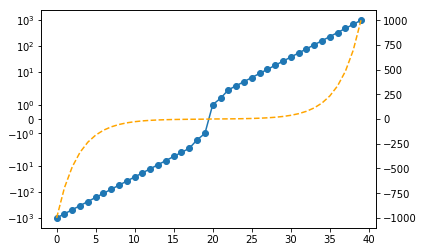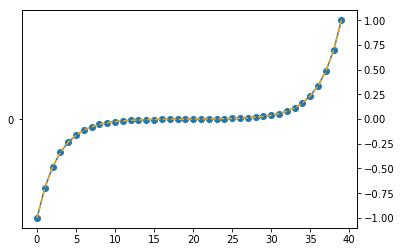# [Matplotlib-users] symlog iffy when range max is below 1e0=1

Hi,

I have a problem using ‘symlog’ when the range maximum is below 1e0=1. Perhaps I’m misusing it, possibly the linthresh option.

Sample code:
import numpy as np
import matplotlib as mpl
import matplotlib.pyplot as plt

def test(start):
linthresh = 10**(start+1)
tmp = np.logspace(start, start + 3, 20)
tmp = np.hstack((-tmp[::-1], tmp))

fig, ax = plt.subplots()
ax.plot(tmp, marker=‘o’)
ax.set_yscale(‘symlog’, linthresh=linthresh)
ax1 = ax.twinx()
ax1.plot(tmp, c=‘orange’, ls=’–’)

test(0) # OK, linear scale and symlog scale look different
test(-3) # iffy, linear scale and symlog scale look the sameIt turns out for yscale, I need to use linthreshy not linthresh. Problem solved.

···

On Tue, Sep 22, 2020 at 11:45 PM Liang Wang <frank0734@gmail.com> wrote:

Hi,

I have a problem using ‘symlog’ when the range maximum is below 1e0=1. Perhaps I’m misusing it, possibly the linthresh option.

Sample code:
import numpy as np
import matplotlib as mpl
import matplotlib.pyplot as plt

def test(start):
linthresh = 10**(start+1)
tmp = np.logspace(start, start + 3, 20)
tmp = np.hstack((-tmp[::-1], tmp))

fig, ax = plt.subplots()
ax.plot(tmp, marker=‘o’)
ax.set_yscale(‘symlog’, linthresh=linthresh)
ax1 = ax.twinx()
ax1.plot(tmp, c=‘orange’, ls=’–’)

test(0) # OK, linear scale and symlog scale look different
test(-3) # iffy, linear scale and symlog scale look the same